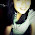## Monday, April 05, 2010

### [Science Form 3] Units of Electricity

Volts (V)
• Voltage can be compared to the pressure of water in a hose. The higher the pressure, the faster the water will flow through the hose.

Similarly, the higher the voltage of electricity, the faster it will flow from the source of the electricity to an end user.

A volt, or the unit of voltage, is a common electrical measurement. It is used, for example, to discuss the transmission of electricity from power plants to end users.

Ohms
• Ohm measure resistance and can be compared to the diameter measurement of a hose. A smaller diameter hose will allow less water to flow through than a larger diameter hose.

Similarly, a thinner wire increases resistance, causing a lesser amount of electricity to be transmitted because it is encountering resistance in the wire.

To reduce resistance, certain metals, such as copper, which allows electrons to flow easily, are used to conduct electricity.

Amperes (A)
• Amperes can be compared to the volume of water that flows through a hose. The volume of water that flows past a certain point in a specific amount of time can be measured.

Similarly, amperes measure the number of electrons that pass a specific point within a certain amount of time (rate of current) in a circuit.

One ampere is equivalent to approximately 6.25 X 1018 electrons passing by a specific point in one second.

The rate of the electric current is dependent upon the voltage and resistance. A circuit with high voltage and low resistance will have more amperes (greater number of electrons passing through the circuit) than a circuit with low voltage and higher resistance. The former example has more power than the latter.

It is important to measure the amount of amperes in a circuit because it can help calculate the amount of power consumption or output of a circuit.

Ohm's Law
1. Ohm's Law is used to describe the mathematical relationship between electric current, voltage and resistance.

2. We can arrange Ohm's Law depending on which component we are trying to find out.
• I = V/R
• V = I x R
• R = V/I

3. All these variations of Ohm's Law are mathematically equal to one another...
Measuring of;
• Current (I)
• Method: Ammeter
Unit= Amperes (A)
# The ammeter is connected in series to a circuit. The movement of the ammeter needle shows that current is flowing in the circuit.

• Voltage (V)
• Method: Voltmeter
Unit: Volts (V)
# The voltmeter is connected in parallel to a circuit when measuring voltage.

• Resistance
• Method: Resistor
Unit: Ohm
# The higher the resistance, the bigger the ohm value in a resistor.

1.FYI RESISTANCE IS MEASURED WITH AN OHMMETER NOT WITH A RESISTOR (UNLESS YOU USE A SHUNT).

2.3.cikgu,terima kasih ye
i hope i can score my science well

## Nota Terkini

• - Sumber-sumber tenaga yang terdapat di dunia ini adalah boleh dikelaskan kepada dua kumpulan, iaitu: 1. Sumber tenaga yang boleh diperbaharui. 2. Sumb...
• - *Hukum-hukum Indeks – Hukum 1* *am x** an= am+n* dimana *a*, *m* dan *n* adalah nombor nyata. *Pembuktian Hukum 1* *Contoh 1* Cari nilai bagi yang ber...
• - Formula am sabun dapat diwakili dengan RCOONa untuk garam natrium atau RCOOK untuk garam kalium dengan R sebagai kumpulan alkil yang berantai panjang. Co...
• - *Kepupusan haiwan* (animal extinction) bermaksud *lenyapnya* atau *sudah tiada lagi* sesuatu haiwan itu di muka bumi ini. Kewujudan haiwan-haiwan tersebut ...
• - Apabila suatu voltan, *V*, dikenakan merentasi katod dan anod suatu senapang elektron, tenaga elektrik yang dibekalkan kepada setiap elektron ialah *eV*, d...